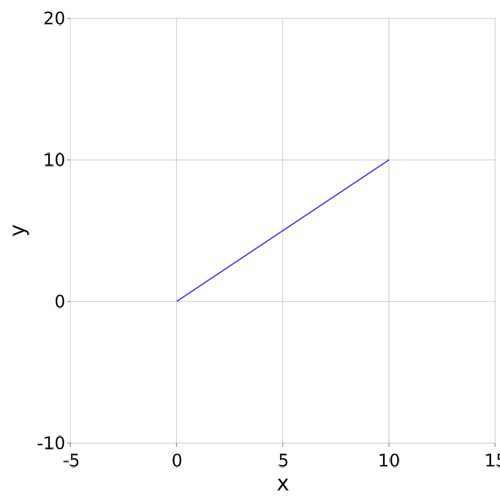```

using AbstractPlotting

x = range(0, stop = 10, length = 40)
y = x
#= specify the scene limits, note that the arguments for FRect are
x_min, y_min, x_dist, y_dist,
therefore, the maximum x and y limits are then x_min + x_dist and y_min + y_dist
=#
limits = FRect(-5, -10, 20, 30)

scene = lines(x, y, color = :blue, limits = limits)

```